Courses

# Test: Ratio And Proportion, Indices, Logarithms - 1

## 40 Questions MCQ Test Quantitative Aptitude for CA CPT | Test: Ratio And Proportion, Indices, Logarithms - 1

Description
This mock test of Test: Ratio And Proportion, Indices, Logarithms - 1 for CA Foundation helps you for every CA Foundation entrance exam. This contains 40 Multiple Choice Questions for CA Foundation Test: Ratio And Proportion, Indices, Logarithms - 1 (mcq) to study with solutions a complete question bank. The solved questions answers in this Test: Ratio And Proportion, Indices, Logarithms - 1 quiz give you a good mix of easy questions and tough questions. CA Foundation students definitely take this Test: Ratio And Proportion, Indices, Logarithms - 1 exercise for a better result in the exam. You can find other Test: Ratio And Proportion, Indices, Logarithms - 1 extra questions, long questions & short questions for CA Foundation on EduRev as well by searching above.
QUESTION: 1

### Choose the most appropriate option (a) (b) (c) or (d) The inverse ratio of 11 : 15 is

Solution:

The inverse ratio of 11:15 is 15:11

QUESTION: 2

### The ratio of two quantities is 3 : 4. If the antecedent is 15, the consequent is

Solution:

Let the numbers be 3x and 4x
given 3x= 15
x= 5
therefore 4x= 4*5= 20...

So, the consequent is 20

QUESTION: 3

### The ratio of the quantities is 5 : 7. If the consequent of its inverse ratio is 5, the antecedent is

Solution:

The ratio of two quantities is 5:7
The inverse ratio is 7:5
The antecedent is 7

QUESTION: 4

The ratio compounded of 2 : 3, 9 : 4, 5 : 6 and 8 : 10 is

Solution:

2×3×9×4×5×6×8×10 = 720:720 which gives 1:1

QUESTION: 5

The duplicate ratio of 3 : 4 is

Solution:

Given ratio is 3:4

since duplicate ratio is by a^2 :b^2

then we have 9:16

QUESTION: 6

The sub duplicate ratio of 25 : 36 is

Solution: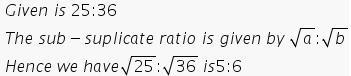QUESTION: 7

The ratio compounded of 4:9, and the duplicate ratio of 3:4,the triplicate ratio of 2:3 and 9:7 is

Solution:

The duplicate ratio of 3:4 is 3^2/4^2 = 9/16.
The triplicate ratio of 2:3 is 2^3/3^3 = 8/27.

Now we have 4 ratios: 4:9, 9:16, 8:27 and 9:7.

We have to calculate the ratio compounded of all these ratios.

That will be (4 x 9 x 8 x 9)/(9 x 16 x 27 x 7) = 2/21.

QUESTION: 8

The sub triplicate ratio of 8 : 27 is

Solution:

sub triplicate ratio of 8:27 is 3√8:3√27=2:3.

QUESTION: 9

The ratio compounded of 4 : 9 and the duplicate ratio of 3 : 4 is

Solution:

Dupliate ratio of 3:4, will be the square of the ratio, so this will be 9:16
and the compounded ratio will be product of two ratios
4:9 x 9:16 = 1:4 ( 9 will be cancelled and 16 by 4 )

QUESTION: 10

The ratio compounded of 4 : 9, the duplicate ratio of 3 : 4, the triplicate ratio of 2 : 3 and 9 : 7 is

Solution:
QUESTION: 11

The ratio compounded of duplicate ratio of 4 : 5, triplicate ratio of 1 : 3, sub duplicate ratio of 81 : 256 and sub triplicate ratio of 125 : 512 is

Solution: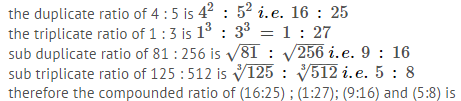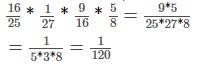QUESTION: 12

If a : b = 3 : 4, the value of (2a+3b) : (3a+4b) is

Solution:

A/b=3/4
So,
[(2 x 3)+(3 x 4)]/[(3 x 3)+(4 x 4)]
(6+12)/(9+16)
18/25

QUESTION: 13

Two numbers are in the ratio 2 : 3. If 4 be subtracted from each, they are in the ratio 3 : 5.The numbers are

Solution:

Let no. 2x and 3x then
(2x-4)/(3x-4)=3/5
10x-20=9x-12
x=8
now, numbers are:2×8=16. &. 3×8=24

QUESTION: 14

The angles of a triangle are in ratio 2 : 7 : 11. The angles are

Solution:

Sum of angle of a triangle is 180 so,
2x+7x+11x=180
20x=180
x=9

now put the value of x than we will get the angles

2x=2*9=18
7x=7*9=63
11x=11*9=99

to verify add them if it is 180 then we are right

99+18+63=180.

QUESTION: 15

Division of Rs. 324 between X and Y is in the ratio 11 : 7. X & Y would get Rupees

Solution:

Here is ratio of division is 11:7 so,
X share = 11a and Y is 7a
total 11a + 7a = 18a
18a = 324
a = 18
X share = 11a= 198 rs
Y share = 7a= 126 rs

QUESTION: 16

Anand earns Rs.80 in 7 hours and Promod earns Rs.90 in 12 hours. The ratio of their earnings is

Solution:

Here find both persons earning in 1 hour.
As Anand earn Rs 80 in 7 hours , so he earn 80 /7 in one hour
And Pramod earn Rs 90 in 12 hours , so he earn 90/12 in one hour .
So the ratio of their earning is Anand : pramod = 807/9012/ =32:21

QUESTION: 17

The ratio of two numbers is 7 : 10 and their difference is 105. The numbers are

Solution:

Since the difference between the two numbers is 105, they are x and x + 105. The ratio of the two numbers is 7/10. So this may be written as

7/10 = x/(x + 105)

7(x + 105) = 10x [Multiply both sides by 10(x + 105) to eliminate the denominators]

7x + 735 = 10x

735 = 3x [Subtract 7x from both sides]

245 = x [Divide both sides by 3]

350 = x + 105

So the two numbers are 245 and 350.

Verification

245/350 = 49/70 = 7/10 [Divide the numerator by 5 then by 7]

QUESTION: 18

P, Q and R are three cities. The ratio of average temperature between P and Q is 11 : 12 and that between P and R is 9 : 8. The ratio between the average temperature of Q and R is

Solution:

P /Q = 11 / 12        P / R = 9 / 8

Q / R =   P/R  /   P/Q    =  9/8  /  11/12  = 9*12 / 8*11  = 27/22

Q : R  = 27 : 22

QUESTION: 19

If x : y = 3 : 4, the value of x2y + xy2 : x3 + y3 is

Solution:

From the given data, we have x = (3/4)y or 4x = 3y

(x2y + xy2) :: (x2 + y2) = xy(x + y)/{(x + y)(x2 - xy + y2) = (xy)/(x2 - xy + y2

Substituting x = (3/4)y from the above,

(x2y + xy2) :: (x2 + y2) = (3y2/4)/(9y2/16 - 3y2/4 + y2) = 12/(9 - 12 + 16) = 12/13

QUESTION: 20

If p : q is the sub duplicate ratio of p–x2 : q–x2 then x2 is

Solution:

P/q = √(p - x^2)/√(q - x^2) p^2/q^2 = (p - x^2)/(q - x^2) Cross multiply (p^2)*(q - x^2) = (q^2)*(p - x^2) p^2*q - p^2*x^2 = q^2*p - q^2*x^2 p^2*q - q^2*p = p^2*x^2 - q^2*x^2 pq(p - q) = x^2*(p^2 - q^2) pq(p - q) = x^2*(p + q)(p - q) pq = x^2*(p + q) X^2 = pq/(p + q)

QUESTION: 21

If 2s : 3t is the duplicate ratio of 2s – p : 3t – p then

Solution:

If given ratio x : y then it's duplicate

ratio = x^2 : y^2

According to the problem given,

2s / 3t = ( 2s -p)^2 / (3t -p)^2

2s( 3t -p )^2 = 3t( 2s - p )^2

2s( 9t^2 - 6tp + p^2 ) = 3t(4s^2 -4sp+ p^2)

18st^2-12spt+2p^2s= 12s^2t-12spt+3p^2t

18st^2-12s^2t= 3p^2t - 2p^2s

6st(3t -2s) = p^2(3t - 2s)

6st = p^2

Therefore ,

p^2 = 6st

QUESTION: 22

If p : q = 2 : 3 and x : y = 4 : 5, then the value of 5px + 3qy : 10px + 4qy is

Solution:

The ratio are as follows

p : q = 2 : 3

Let The value of p = 2 a ,

Let The value of q = 3 a

And

x : y = 4 : 5

Let The value of x = 4 b

Let The value of y = 5 b

Now, According to question

Let 5 p x + 3 q y : 10 p x + 4 q y  = m :n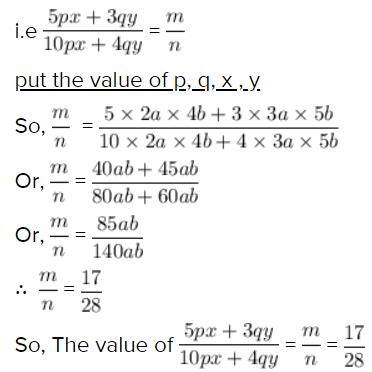QUESTION: 23

The number which when subtracted from each of the terms of the ratio 19 : 31 reducing it to 1 : 4 is

Solution:

19-x/31-x =1/4
76-4x =31-x
-3x = -45
x=45/3
x÷15
so that number is 15.

QUESTION: 24

Daily earnings of two persons are in the ratio 4:5 and their daily expenses are in the ratio 7 : 9. If each saves Rs. 50 per day, their daily incomes in Rs. are

Solution:

Let the monthly income of one person be 4x and that of the other be 5x

Let the monthly expenses of one person be 7y and that of other be 9y

ATQ

4x-7y=50-(1)-*5=20x-35y=250

5x-9y=50-(2)-*4=20x-36y=200

=y=50

from 1

4x-7*50=50

4x=50+350

4x=400

x=100

Monthly income of one person=4x=4*100=400

Monthly income of the other person=5x=5*100=500

QUESTION: 25

The ratio between the speeds of two trains is 7 : 8. If the second train runs 400 Kms. in 5 hours, the speed of the first train is

Solution:

Speed of second train = Distance travelled / time = 400 /5 = 80 km/hr
Ratio of speeds = 7 :8 = (7/8) :1
If speed of second train is x, then the speed of first train is 7x/8
7x/8 = 7 x 80/8 = 70
So, speed of first train is 70 km/hr

QUESTION: 26

The fourth proportional to 4, 6, 8 is

Solution:

For proportions; the product of the internal terms equals the product of the externals.

Therefore,

=> 4 : 8 = 6: x

=> 4x = 48

=> x = 12.

QUESTION: 27

The third proportional to 12, 18 is

Solution:

Let the third proportion be x

12 : 18 = 18 : x

x = 18*(18/12)

x = 18*(3/2)

x = 54/2

x = 27

QUESTION: 28

The mean proportional between 25, 81 is

Solution: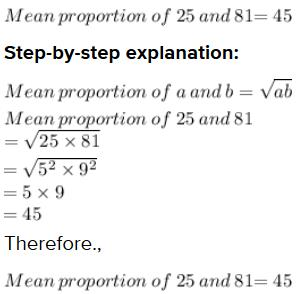QUESTION: 29

The number which has the same ratio to 26 that 6 has to 13 is

Solution:

The ratio of the number similar to 6∶13 is 12∶26

Given:

One ratio of the number is 6 : 13 which becomes 6/13

To find:

The another ratio of number similar to 6 : 13

Solution:

Let the unknown number be x

Given that another ratio of the number is x: 26 which becomes x/26

Given that the two ratios are similar, equating the two terms, we get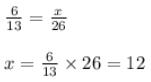Hence the ratio of the number similar to 6∶13 is 12∶26

QUESTION: 30

The ratio of the present age of father to that of son is 7:2. After 10 years their ages will be in the ratio of 9:4. The present ages of the father is

Solution:

Let father age be X and son age be Y. Then
X/Y = 7/2
2X = 7Y ..... (1)
After ten years ,
(X+10)/(Y+10) = 9/4
4(X+10) = 9(Y+10)
4X + 40 = 9Y + 90
4X = 9Y + 50
2×2X = 9Y + 50

putting the value of 2X in equation 1

2×7Y = 9Y + 50
14Y = 9Y + 50
5Y = 50
Y = 10
Son age is 10 years .

putting the value of Y in equation 1

2X = 7Y
X = 7/2Y
X = 7/2×10
X = 70/2
X = 35
Father age is 35.

QUESTION: 31

If four numbers 1/2, 1/3, 1/5, 1/x are proportional then x is

Solution: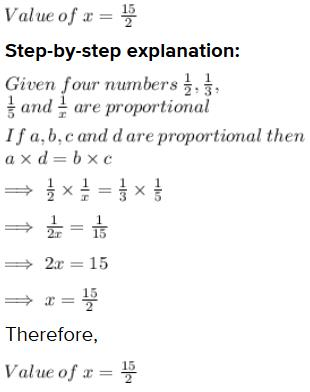QUESTION: 32

The mean proportional between 12x2 and 27y2 is

Solution: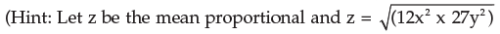QUESTION: 33

If A = B/2 = C/5, then A : B : C is

Solution:

I think you take all in respect to A and cut it out
∴ a= a
b = 2a
c= 5a
a:b:c = 1:2:5

QUESTION: 34

If a/3 = b/4 = c/7, then a+b+c/c is

Solution: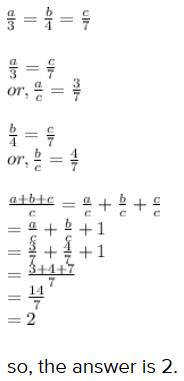QUESTION: 35

If p/q = r/s = 2.5/1.5, the value of ps:qr is

Solution:

Solution:

p/q = r/s = 2.5/1.5 = 5/3

Now, ps:qr = ps/qr = 5 x 3/3 x 5 = 1:1

QUESTION: 36

If x : y = z : w = 2.5 : 1.5, the value of (x+z)/(y+w) is

Solution:

x/y = 5/3

x = 5/3 y

z/w = 5/3

z = 5/3 w

x + z = 5/3 y + 5/3 w

= 5/3( y + w)

(x + z)/(y + w) = 5/3

QUESTION: 37

If (5x–3y)/(5y–3x) = 3/4, the value of x : y is

Solution:

( 5x - 3y ) / ( 5y - 3x ) = 3/4
Cross multiplying the numbers in the left and right ,
4 (5x - 3y) = 3 (5y - 3x)
Opening the brackets ,
20x - 12y = 15y - 9x
Grouping like terms to one side ,
20x + 9x = 15y + 12y
29x = 27y
→ 29 * x = 27 * y
→ x / y = 27 / 29
→ x:y = 27:29

QUESTION: 38

If A : B = 3 : 2 and B : C = 3 : 5, then A:B:C is

Solution:

The obtained ratio for A: B: C is 9 : 6 : 10

To find:

A: B: C = ?

Solution:

Given :

A: B = 3: 2 and B: C = 3: 5

To compare two ratios, we need to make one of the ratios equal. Here we had B term in both the ratios, so we are making two terms equal by making B equal.

Multiplying A: B i.e. 3: 2 by 3

and B: C i.e. 3: 5 by 2 to bring the value of B at par, we get

A: B = 9: 6 & B: C = 6: 10

Hence, the value of A: B: C is obtained to be 9 : 6 : 10

QUESTION: 39

If x/2 = y/3 = z/7, then the value of (2x–5y+4z)/2y is

Solution: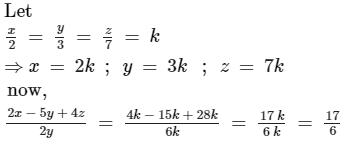QUESTION: 40

If x : y = 2 : 3, y : z = 4 : 3 then x : y : z is

Solution:

Making y same, we get

x:y = 8 :12 (multiplied by 4)

y:z = 12:9  (multiplied by 3)

x:y:z = 8:12:9